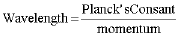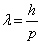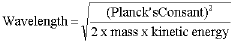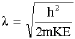DeBroglie Wavelength

Louis de Broglie was the first person to establish an equation for the relationship between an electron’s momentum and its wavelength. He concluded that:where h is a number called Planck’s constant (named after Max Planck) and is equal to 6.63 x 10-34 J × s or 4.14 x 10-15 eV × s.

When we observe electron diffraction, the electrons’ kinetic energy is easier to measure than their momentum, so we write the de Broglie wavelength asorThis equation is consistent with our results in the previous activity — as the energy increases the wavelength decreases.